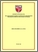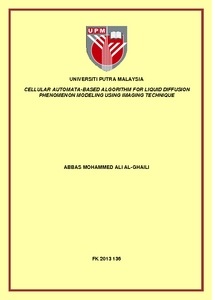# Cellular Automata-based Algorithm for Liquid Diffusion Phenomenon Modeling using imaging technique

## Citation

Al-Ghaili, Abbas Mohammed Ali (2013) Cellular Automata-based Algorithm for Liquid Diffusion Phenomenon Modeling using imaging technique. PhD thesis, Universiti Putra Malaysia.

## Abstract

Recently, the prediction of the dynamical behavior of Liquid Diffusion Phenomenon (LDP) has been used in many applications especially in physical and biological fields. Many models have been proposed to predict the LDP behavior, but most of them require complex mathematical calculations causing computation time consumption. This thesis proposes a dynamical behavior prediction algorithm using Cellular Automata (CA) to model the LDP. A real liquid diffusion phenomenon is recorded whereas the observed images are later extracted for comparing purpose with the predicted phenomenon. First, a mathematical method is proposed in order to track and then analyze the real diffusion behavior. This method has used thousands of original images. Then, thousands of images, as the same number of original images, are created by the CA-based algorithm. In this study, the diffusion speed of the predicted LDP is also computed by using a mathematical proposed algorithm which is the Diffusion Speed Algorithm (DSA). Finally, three benchmark strategies are used in order to compare the predicted images to the original images, which are:pixel intensity, Region-of-Diffusion (ROD) area, and ROD shape. The experiments of this thesis are divided into original and predicted images. The original images are classified into three groups based on the temperature used, which are: ±18 °C, ±24 °C, and ±30 °C. Each temperature-based experiment contains five levels of the height of droplets source. The diffusion time has been equal to 32 seconds with 15 fps comprising 480 images per each experiment. On the other hand,the predicted images are similarly classified. There will be 15 predicted experiments created by the proposed CA algorithm. The whole predicted images are compared to their corresponding original ones. Under the experiments samples, there are 30 processed experiments comprising 14400 original and predicted images. The obtained results show that the averaged similarity percentage is equal to 94.4%. Additionally,the average computation time needed to complete processing a single experiment is 1.3 second. The result obtained from the proposed LDP model compared to other competitive LDP models has higher accuracy and less computation time. The results also show that the proposed LDP model is about 15 times faster than a neural network-based model. A detailed study to explore the effects and relationships between the model‟s parameters such as temperature and liquids‟ viscosity has been performed. The results showed that there is a direct relationship between the temperature and the diffusion speed.Preview
PDF
FK 2013 136RR.pdfView Item